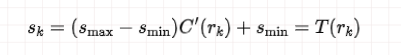﻿ Python学习之直方图均衡化原理详解_python_脚本之家
python# Python学习之直方图均衡化原理详解

## 2.线性灰度变换▲图2.1 线性灰度变换▲图2.2 图像对比度（左为弱对比度，右为强对比度）

## 3.直方图均衡化▲图3.1 图像对比度示例▲图3.2 直方图均衡化原理## 4.代码实战

```# 计算累计分布函数
def C(rk):
# 读取图片灰度直方图
# bins为直方图直方柱的取值向量
# hist为bins各取值区间上的频数取值
hist, bins = np.histogram(rk, 256, [0, 256])
# 计算累计分布函数
return hist.cumsum()
```
```# 计算灰度均衡化映射
def T(rk):
cdf = C(rk)
# 均衡化
cdf = (cdf - cdf.min()) * (255 - 0) / (cdf.max() - cdf.min()) + 0
return cdf.astype('uint8')
```

```import numpy as np
import cv2 as cv
from matplotlib import pyplot as plt

# 计算累计分布函数
def C(rk):
# 读取图片灰度直方图
# bins为直方图直方柱的取值向量
# hist为bins各取值区间上的频数取值
hist, bins = np.histogram(rk, 256, [0, 256])
# 计算累计分布函数
return hist.cumsum()

# 计算灰度均衡化映射
def T(rk):
cdf = C(rk)
# 均衡化
cdf = (cdf - cdf.min()) * (255 - 0) / (cdf.max() - cdf.min()) + 0
return cdf.astype('uint8')

# 读取图片
# 将二维数字图像矩阵转变为一维向量
rk = img.flatten()

# 原始图像灰度直方图
plt.hist(rk, 256, [0, 255], color = 'r')
cv.imshow("原图像",img)

# 直方图均衡化
imgDst = T(rk)[img]
cv.imshow("直方图均衡化后的图像",imgDst)
plt.hist(imgDst.flatten(), 256, [0, 255], color = 'b')

plt.show()
```▲图4.1 直方图均衡化效果(灰度直方图)▲图4.2 均衡化前▲图4.3 均衡化后